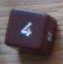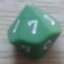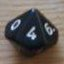Complex multi-die "choose"-ing

## Complex multi-die "choose"-ing

 Fri Mar 21 02:15:10 2014   by   Jake Firstly I would like to thank you again for developing such a very robust and powerful tool; there have been very few problems or instances that Troll hasn't been able to model for me, or at least give me insight into. Below is a problem I am working on that I haven't been able to easily model as of yet:    I am trying to turn the sum of dice rolls into tabular data. Now if it was a single die with flat probablities then I could use the “choose” command [ie: choose{a,b,c,d,e,f,g} easily represents rolling a D7 and substituting the 7 different results with my chosen letter.], however I am looking to work the the results of a multi-die sum. [ie: choose{a,b,c,d,e,f,g} where you add 2d4 and get one of the 7 different results from the 7 different sums].    Since I'm primarily modeling 2d4s I am willing to write out a lengthy choose command set [ie: choose{a,b,b,c,c,c,d,d,d,d,e,e,e,f,f,g}] to get the equivalently modeled results. But if/when I need to use more dice, higher sides, mixed types; writing out a longhand list to “brute-force” a nonlinear choose probability gets quickly exhaustive (and easily prone to errors).    What I am ultimately looking to do is roll 2d4+X (“X” being a constant between 0 & 17) and equate the resulting sum to a fixed table of 24 values. This sort of “floating” of the sum makes even writing the simple chose function out longhand cumbersome as I would need to write 18 slightly different versions of the probabilistic function out (one for each possible value of X)    Can you offer any advice or way I  could model this situation with troll? (hopefully  I'm not overlooking something obvious lol) I was thinking a variant of the “choose” command where the selections could be chosen with weighted probability instead of equal probability might be useful for myself and others; but I can see how it might be a pain to write or implement hehe. Thanks again Fri Mar 21 10:17:12 2014   by   Torben A simple way to get weighted probabilities is something like`choose{a,2#b,3#c,4#d,3#e,2#f,g}`corresponding to the 16-entry choose you listed above.As for the 24-entry table, you can get the n'th lowest element of a collection by `max (least n c)`, where `c` is the collection.  So you could handle the 2d4+X lookup by`max (least (d4+d4+X) {....})`where the dots are replaced by the 24 values you want (using the shorthand above).  Note that, since you get the n'th lowest element rather than the n'th element, this only works if your intended table is sorted.  If you want a table with values in an order that is neigter ascending nor descending, you would need to use a nested if-then-else for the table lookup, which is rather cumbersome. Fri Mar 21 23:20:28 2014   by   Jake Perfect! Thank you :) Fri Mar 21 23:20:38 2014   by   Jake Perfect! Thank you :)